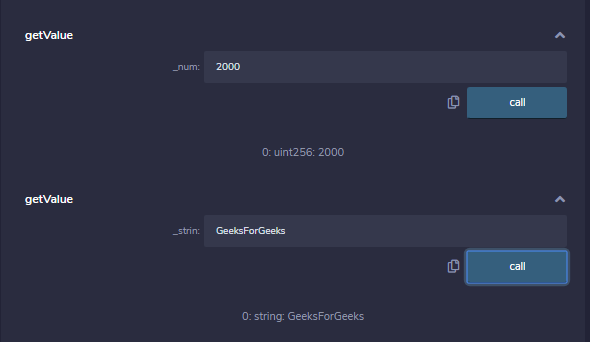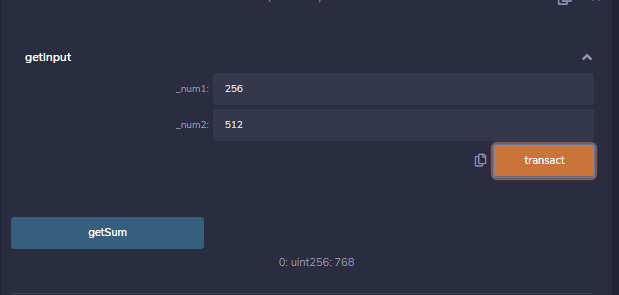Related Articles

# Solidity – Polymorphism

• Last Updated : 13 Jul, 2020

Polymorphism is an ability to process data in more than one form. Like any other programming language Solidity also supports polymorphism. Solidity supports two types of polymorphism, Function Polymorphism, and Contract Polymorphism.

### Function Polymorphism

Function Polymorphism is also known as method overloading. In function polymorphism, multiple functions are declared having the same name within the same contract or inheriting contract. Functions differ on the basis of the number of parameters or parameter datatypes. Declaration of function cannot be overload by functions that differ only in return type.

Example: In the below example, the contract methodOverloading defines two functions with the same name but different argument lists to demonstrate function polymorphism.

## Solidity

 `// Solidity program to demonstrate``// Function Polymorphism``pragma solidity ^0.5.0;`` ` `// Contract definition``contract` `methodOverloading {``     ` `    ``// Function to get value ``    ``// of the string variable``    ``function getValue(``      ``string memory _strin) ``public` `pure returns(``      ``string memory){``        ``return` `_strin;``    ``}`` ` `    ``// function to get value of ``    ``// the unsigned integer variable ``    ``function getValue(``      ``uint _num) ``public` `pure returns(``      ``uint){``        ``return` `_num;``    ``}``}`

Output :### Contract Polymorphism

Contract polymorphism means using multiple contract instances interchangeably when they are related to each other by using inheritance. This helps in calling the child contract functions using the instance of the parent contract.

Example: In the below example, the contract parent is derived by the child contract child, to demonstrate contract polymorphism. ContractPolymorphism is the driver contract.

## Solidity

 `// Solidity program to demonstrate ``// Contract Polymorphism``pragma solidity >=0.4.22 <0.6.0;  ``  ` `// Contract definition ``contract` `parent{  ``     ` `    ``// Internal state variable  ``    ``uint internal sum;  ``     ` `    ``// Function to set the value of ``    ``// internal state variable sum  ``    ``function setValue(``      ``uint _num1, uint _num2) ``public` `{  ``        ``sum = _num1 + _num2;``    ``}  `` ` `    ``// Function to return a value 10``    ``function getValue(``    ``) ``public` `view returns(uint) {  ``        ``return` `10;  ``    ``}  ``}  `` ` `// Defining child contract   ``contract` `child is parent{  ``     ` `    ``// Function getValue overloaded ``    ``// to return internal state ``    ``// variable sum defined in the ``    ``// parent contract``    ``function getValue(``    ``) ``public` `view returns(uint) {  ``        ``return` `sum;  ``    ``}  ``}  `` ` `// Defining calling contract  ``contract` `ContractPolymorphism {  ``     ` `    ``// Creating object``    ``parent pc = ``new` `child();   ``       ` `    ``// Function to set values ``    ``// of 2 unsigned integers``    ``function getInput(``      ``uint _num1, uint _num2) ``public` `{  ``        ``pc.setValue(_num1, _num2);  ``    ``} `` ` `    ``// Function to get value of ``    ``// internal state variable sum ``    ``function getSum(``    ``) ``public` `view returns(uint){``        ``return` `pc.getValue(); ``    ``}``}`

Output :My Personal Notes arrow_drop_up